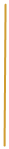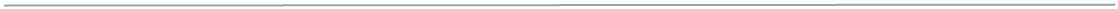High School
Math Curriculum

ALGEBRA

1) Expressions and Equations
A. Variables and expressions;
I. Writing verbal expression for algebraic expression
II. Writing algebraic expression for verbal expression,
B. Order of operation;
C. Distributive property;
D. Equation;
I. Solving the equation with two variables given a domain.
2) Linear equation:
A. Translate sentence into equation;
B. Solving one-step equation by using mathematical operations;
C. Solving multi - step equation by using mathematical operations;
D. Solving equations with the variables on each side; involving grouping
symbols;
E. Evaluate absolute value expression;
F. Solving absolute value equations.
3) Representing linear equation
A. Graphing linear equation;
B. Solving linear equation by graphing;
C. Slope of line;
D. Writing and graph linear equation in slope - intercept form;
E. Model real world data with equations in slope-intercept form;
F. Creating equations of lines in standard form; point-slope form and
different forms;
G. Parallel and perpendicular lines.
4) Linear inequalities
A. Solving linear inequalities by using different mathematical operation;
B. Solving compound inequalities;
C. Solving and graph absolute value inequalities;
D. Inequalities in two variables.
5) Systems of linear equations and inequalities
A. Solving systems of linear equation by graphing, substitution and
elimination;
B. Applying systems of equation to solve real life problems;
C. Matrix equations - Gaussian elimination.
6) Polynomials
A. Multiplying and dividing polynomials;
B. Mathematical operation on polynomials.
A. Factoring monomials - the Greatest Common Factor;
B. Solving the quadratic equation using the zero product property;
D. Solving the quadratic equation using the square root property and
graph;
8) Rational expression
A. Simplifying rational expression using common factor to reduce;
B. Mathematical operation on rational expression;
C. Determined the Least Common Denominator.
9) Applying fractions
A. Ratios and proportions;
B. Percent - solving real-world problems;
C. Solving equation with fractions;
D. Negative exponents - simplifying the expression and using scientific
notation.
A. Identifying properties of rational number;
B. Rational and irrational square roots;
C. Mathematical operation on square roots;
D. Using the Power Property of Equality to solve the radical equation.
11) Pythagorean’s theorem
A. Solving real life problems involving right triangle.
12) Relation and function
A. Determined the domain and range of function;
B. Function one-to-one and onto;
C. Graph absolute value and quadratic function;
D. Identifying the roots and sketch higher degree polynomial function;
E. Perform operation with functions;
F. Evaluate the composition of function;
G. Find and graph the inverse of function;
H. The equation of circle.
B. Operation with complex numbers;
C. Sum and product of the roots of quadratic equations - Vieta's formulas;
D. Graph system of equations involving quadratic equations, line, circles
and inequalities.
14) Exponential function
A. Evaluate expression with exponents;
B. Graph exponential function;
C. Solve equation with exponents;
D. Solve exponential equation and inequalities.
15) Logarithmic functions
A. Find the inverse of an exponential function;
B. Apply logarithmic relationship;
C. Solve common logarithmic problems;
D. Solve exponential equations requiring logarithms.

GEOMETRY

1) Fundamental concepts and pattern
A. Using inductive and deductive reasoning to explore and recognize
geometric pattern;
B. Distinguish between inductive and deductive reasoning;
C. Identify and apply basic definitions and postulates of geometry;
D. Statement in if-then form and their converses;
E. Segment construction congruent to a given segment;
F. Use the number line to calculate distance;
G. Midpoint of given statement;
H. Graph point and lines in the coordinate plane.
2) Angles, parallel and perpendicular lines
A. Parts of an angle;
B. Angles classification by their measure;
C. Angle relationship - complementary, vertical, congruent - identifying
and applying;
D. Define and apply perpendicular lines;
E. Construct the bisector of a given angle and an angle congruent to a
given angle;
F. Construct a perpendicular line;
I.  To a line at a given point,
II. From a given point to a line.
G. Construct the perpendicular bisector of given segment;
H. Parallel line and plans - difference;
I. Definition of slope and finding slope of parallel and perpendicular
line to given.
3) Triangle
A. Triangle classification by sides and angles;
B. Applying the Triangle angle sum theorem;
C. Applying the Isosceles triangle theorem and Exterior angle theorem;
D. Identifying and named corresponding part of congruent polygons;
E. Prove triangle congruence by AAS, SAS, ASA, SSS theorems;
F. Median and altitude of triangle;
G. Pythagorean Theorem - applying and its conversions,
H. Distance between two points - distance formula.
4) Polygons
A. Characteristics of quadrilaterals - parallelogram, rectangles, rhombi,
trapezoid;
B. Regular and non-regular polygons;
C. Determined the area and perimeter of quadrilaterals using appropriate
theorems and formula;
D. Measure of interior and exterior angles of regular and non-regular
polygons.
5) Ratios, proportion and similarity
A. Identification of similar polygons and corresponding parts of similar
polygons;
B. Applying proportions to problems using similar figures;
C. Prove triangles similar using AA, SAS, SSS similarity theorems;
D. Relationship between parallel lines and proportional segments in
triangles;
E. Line and rotation symmetry;
F. Determined and construct geometric transformation including
reflection,
rotation, translation and dilatation;
G. Construct transformation of figure on coordinate plane.
6) Circles and inscribed polygons
A. Area and circumference of circle;
B. Applying relationship among inscribed and central angles and arcs of
circles;
C. Calculate arc length and area of sector;
D. Problems with inscribed and circumscribed polygons;
E. Identify and apply properties of tangents and secants to circles.
7) Surface area and Volume
A. Identify and classify solid figure;
B. Volume and surface area of solid figure;
C. Comparison of linear dimensions, perimeter, surface area, and volume
of similar figures;
D. Explore net of 3 dimensional figures.
8) Right triangle and trigonometry
A. Solve problems involving right triangle and special right triangle
(45-45-90; 30-60-90);
B. Finding the missing part of triangle (angle, side) by using
trigonometric functions.

TRIGONOMETRY

1) Trigonometric functions
A. Right angle trigonometry;
B. Relationship between trigonometric ratios in the unit circle;
C. Reciprocal trigonometric function;
D. Exact value chart;
E. Reference angles.
2) More trigonometric functions
A. Conversion between degree and radians;
B. Radian measure to find value of trigonometric functions;
C. Pythagorean identities;
D. Domain and range of trigonometric functions;
E. Inverse trigonometric functions;
F. Cofunctions identity.
3) Graphs of trigonometric functions
A. Graph sine and cosine on the coordinate plane;
B. Amplitude, frequency, period and phrase shift;
C. Graph tangent function;
D. Graph reciprocal trigonometric functions;
E. Graph inverse trigonometric functions.
4) Trigonometric identity
A. Prove trigonometric identity;
B. Sum and difference formula for sine, cosine and tangent;
C. Double angle formulas for sine, cosine and tangent;
D. Half angle formulas for sine, cosine and tangent.
5) Trigonometric equation
A. Solve first degree trigonometric equation;
B. Solve second degree trigonometric equations using factoring and the
C. Solve trigonometric equation using substitution.
6) Trigonometric application
A. Use law of cosines;
B. Use law of sines;
C. Find the area of triangle.
7) Sequence and Series
A. Determined the difference between an arithmetic and geometric
sequences;
B. Determined the difference between an arithmetic, geometric, and
infinite series;
C. Use sigma notation.
8) Statistics
A. Gather and organize data;
B. Find measures of central tendency;
C. Find standard deviation and variance;
D. Recognize and use the normal distribution;
E. Bivariate statistics and the correlation coefficient;
F. Distinguish between exponential, logarithmic, power, quadratic, cubic,
and linear regression.
9) Probability and binomial theorem
A. Counting principles, combination and permutation;
B. Calculate probability using at least and at most;
C. The normal approximation to the binomial distribution;
D. Apply the binomial theorem.